# Polygons

A polygon is a 2 dimensional figure that has the following attributes:

• It is formed by three or more segments called sides (cannot be curved)
• Each side intersects exactly two other sides at each of its endpoints (cannot intersect in the interior)
• Each endpoint is a vertex of the polygon (cannot be open)

Determine if the figure is a polygon.

A B C D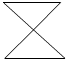A. Yes B. No; it is open C. No; it is curved D. No; it intersects in the interior

Polygons can either be convex or concave.

Examples of convex.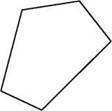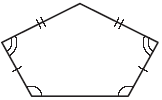Examples of concave.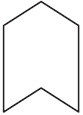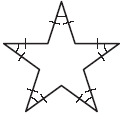Classifying Polygons:

You can classify polygons by the number of sides that they have.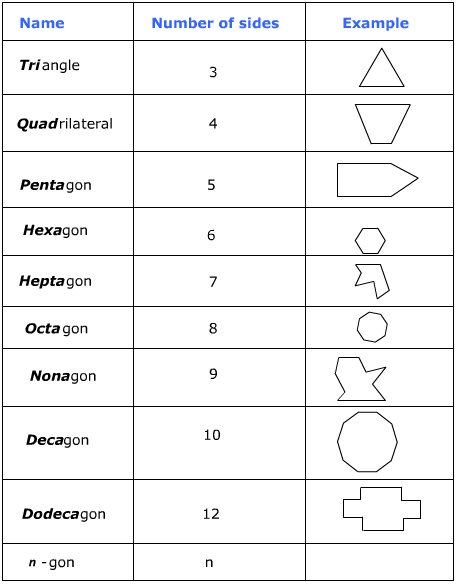There are three special terms for polygons: equilateral, equiangular and regular.

• Equilateral means all sides are equal
• Equiangular means that all angles are equal
• Regular means that all the sides and the angles are equal (equilateral AND equiangular)

Let's look at some examples.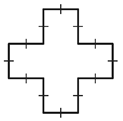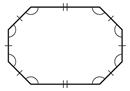Equilateral (all sides equal) Equiangular (all angles are equal)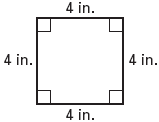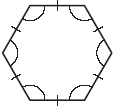Regular (all sides and angles are equal) Regular (all sides and angles are equal)

Polygons are plane figures (2 dimensional flat figures) that are formed by sides that do not have curves or intersect in the interior. Polygons can be classified according to the number of sides. Their form can either be convex or concave. Remember that concave has a "caved in" look. If all the sides are equal then it is equilateral. If all the angles are equal then it is equiangular. If the polygon is both equilateral and equiangular then it is regular.

 Related Links: Math Geometry Topics Supplementary Angles Complementary Angles Regular Polygons Regular and Irregular Polygons Area of Polygons Perimeter of Polygons Interior Angles of a Polygon Exterior Angles of a Polygon

To link to this Polygons page, copy the following code to your site: